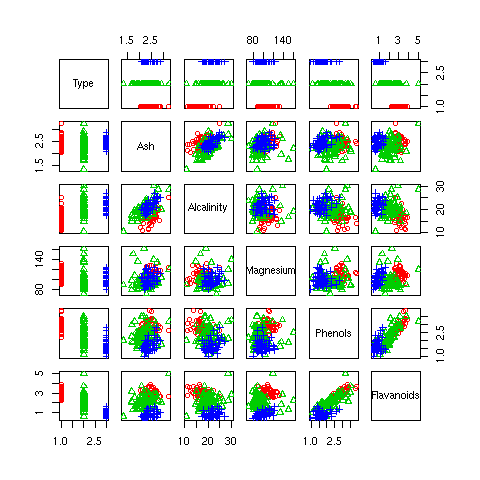DATA MINING
Desktop Survival Guide
by Graham Williams## Multi-Dimension Scatterplot

For data with multiple dimensions, plot will decide on a multi-dimensional scatterplot. This produces a scatterplot for each pair of variables, with the variable names identified in the diagonal. Each plot is a scatterplot of the data for the variable in the column by the variable in the row. The upper right triangle of the scatterplot is the mirror image of the lower left triangle of the scatterplot--with the axes swapped. Although this results in repeated information, it is visually effective since it is possible to scan all plots for one variable either vertically or horizontally, rather than having to turn corners.

Once again, we use different symbols (and colour) to highlight the distribution of Type across each plot, borrowing (but not showing) the appropriate code from the scatterplot example of See Section 32.2.1. The plot is also limited to just the first six variables, to avoid too much clutter. Note that the scatterplot of Section 32.2.1 is also included.```plot(wine[,c(1, 4:8)], col=colours, pch=iType) ```

http://rattle.togaware.com/code/rplot-wine-scatterm.R